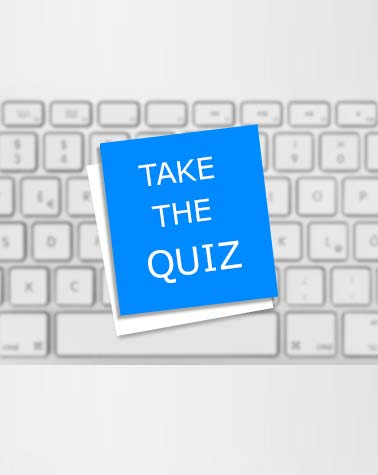# Bank Po Computer Awareness Quiz

35 Questions | Total Attempts: 46SettingsI

Related Topics
• 1.
All of the following are examples of real security and privacy risks EXCEPT:
• A.

Hackers

• B.

Spam

• C.

Viruses

• D.

Identity theft.

• 2.
A process known as ____________ is used by large retailers to study trends.
• A.

data mining

• B.

data selection

• C.

POS

• D.

Data conversion

• 3.
Which of the following is a part of the Central Processing Unit?
• A.

Printer

• B.

Key board

• C.

Mouse

• D.

Arithmetic & Logic unit

• 4.
A(n) ____________ system is a small, wireless handheld computer that scans an items tag and pulls up the current price (and any special offers) as you shop.
• A.

PSS

• B.

POS

• C.

Inventory

• D.

Data mining

• 5.
• A.

Computer aided design

• B.

Computer algorithm for design

• C.

Computer application in design

• D.

All of the above

• 6.
The lowest form of Computer language is called
• A.

BASIC

• B.

FORTRAN

• C.

Machine Language

• D.

COBOL

• 7.
Which of the following is NOT one of the four major data processing functions of a computer?
• A.

Gathering data

• B.

Processing data into information

• C.

Analyzing the data or information

• D.

Storing the data or information

• 8.
Surgeons can perform delicate operations by manipulating devices through computers instead of manually. This technology is known as:
• A.

robotics.

• B.

Computer forensics.

• C.

Simulation.

• D.

Forecasting

• 9.
Memory which forgets every thing when you switch off the power is known as
• A.

Corrupted

• B.

Volatile

• C.

Non-Volatile

• D.

Non-Corrupted

• E.

None

• 10.
The linking of computers with a communication system is called
• A.

Networking

• B.

Pairing

• C.

. Interlocking

• D.

Assembling

• E.

Sharing

• 11.
____________ is the science that attempts to produce machines that display the same type of intelligence that humans do.
• A.

Nanoscience

• B.

Nanotechnology

• C.

Simulation

• D.

Artificial intelligence (AI)

• 12.
____________ is data that has been organized or presented in a meaningful fashion.
• A.

A process

• B.

Software

• C.

Storage

• D.

Information

• 13.
The name for the way that computers manipulate data into information is called:
• A.

Programming.

• B.

processing.

• C.

Storing

• D.

Organizing

• 14.
Computers gather data, which means that they allow users to ____________ data.
• A.

Present

• B.

Input

• C.

Output

• D.

store

• 15.
Which of the following refers to a small, single-site network?
• A.

LAN

• B.

DSL

• C.

RAM

• D.

CPU

• 16.
How many options does a BINARY choice offer
• A.

None

• B.

One

• C.

Two

• D.

It depends on the amount of memory on the computer

• 17.
A collection of program that controls how your computer system runs and processes information is called
• A.

Operating System

• B.

Computer

• C.

Office

• D.

Compiler

• E.

Interpreter

• 18.
Computer connected to a LAN (Local Area Network) can
• A.

Run faster

• B.

Go on line

• C.

Share information and /or share peripheral equipment

• D.

E-mail

• E.

None

• 19.
Computers use the ____________ language to process data.
• A.

Processing

• B.

kilobyte

• C.

Binary

• D.

Representational

• 20.
In the binary language each letter of the alphabet, each number and each special character is made up of a unique combination of:
• A.

Eight bytes

• B.

Eight kilobytes.

• C.

Eight characters.

• D.

Eight bit

• 21.
A ____________ is approximately one billion bytes.
• A.

Kilobyte

• B.

Bit

• C.

Gigabyte

• D.

Megabyte

• 22.
Data can be a number, a word, a picture, or a sound.
• A.

True

• B.

False

• 23.
Currently, the performance of tasks by robots is based on preprogrammed algorithms.
• A.

True

• B.

False

• 24.
Output devices store instructions or data that the CPU processes.
• A.

True

• B.

False

• 25.
The CPU and memory are located on a special circuit board in the system unit called the motherboard.
• A.

True

• B.

False